# Civil Engineering - UPSC Civil Service Exam Questions

36.

Given that 'φ' is the angle of internal friction, 'p' is the safe bearing capacity and γ is the unit weight of soil, the minimum depth of foundation of a masonry footing is given by

 A.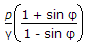B.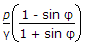C.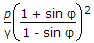D.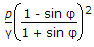Explanation:

No answer description available for this question. Let us discuss.

37.

Which one of the following can be a set of velocity components of a two-dimensional flow ?

 A. u = x + y and v = x2 + y2 B. u = x + y and v = x - y C. u = xy and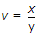D. u = x2 + y2 and v = x2 - y2

Explanation:

No answer description available for this question. Let us discuss.

38.

When is a soil mass said to have entered the solid phase ?

 A. When loss in water is not accompained by a corresponding reduction in the volume of soil mass B. When reduction in the volume of the soil mass is nearly equal to the volume of water lost. C. When the soil mass becomes brittle D. When the soil mass shows a small shearing resistance as the water content is reduced.

Explanation:

No answer description available for this question. Let us discuss.

39.

A centrifugal pump is required to lift 2.8 cu.m.s of water to a height of 7.5 m. If the total loss of head in the pipe system is 0.25 m and the efficiency of the pump is 80%, then the brake horse power will be

 A.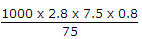B.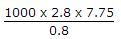C.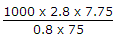D.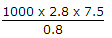Explanation:

No answer description available for this question. Let us discuss.

40.

Which among the following assumptions are made in the design roof trusses ?
1. Roof truss is restrained by the reactions
2. Axes of the members meeting at a joint intersect at a common point
3. Riveted joints act as frictionless hinges
4. Loads act normal to roof surface
Select the correct answer using the codes given below:

 A. 1, 2 and 4 B. 2, 3 and 4 C. 1, 3 and 4 D. 1, 2 and 3